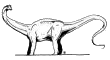Search IntMath
Close

# Polynomial Equations (Equations of Higher Degree)

by M. Bourne

### Historical Solutions...

This section contains some dinosaur mathematics (that is, it is extinct). With software tools, there is no need to churn through pages of algebra solving these things. It is more important to know what it all means and how you can apply it to real problems.This chapter discusses methods for solving higher degree polynomial equations.

In the study of polynomial equations, the most important thing is to understand what "solution of an equation" means. For equations of higher degree, allow for many solutions. The maximum number of solutions you can get is the degree of the polynomial.

After you finish this chapter, you should be able to use a Computer Algebra System to find solutions, either...

Polynomial graph

(a) Graphically, or
(b) Using the CAS directly.

Applications of Root Finding

• modelling
• curve fitting
• instrumentation
• ecological and industrial safety
• industrial maintenance and control problems

## In this Chapter

We begin by defining polynomials and showing how to find roots of a polynomial in 1. Polynomial Functions and Equations »

## Problem SolverThis tool combines the power of mathematical computation engine that excels at solving mathematical formulas with the power of GPT large language models to parse and generate natural language. This creates math problem solver thats more accurate than ChatGPT, more flexible than a calculator, and faster answers than a human tutor. Learn More.